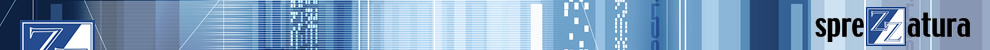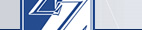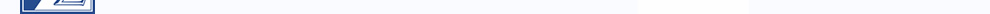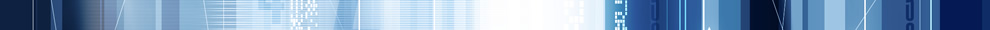In order for this site to work correctly, and for us to improve the site, we need to store a small file (called a cookie) on your computer.# RevMedia

1. Volume 1
2. Volume 2
3. Volume 3
4. Volume 4

### Reader's Forum - Numeric Precision in R/Basic - Hal Wyman

#### R/Basic variables and constants

Variables in R/Basic are untyped as far as the programmer is concerned. But in reality, an R/Basic variable will contain information in one of three forms: a string, an integer, or a floating point number. Variables will be converted among these three formats as necessary. In general, arithmetic operations require that the operands be in one of the numeric type, while concatenation, substring extraction, and the dynamic array operators require operands of string type. (The logical functions BITAND(), etc., require integer format.)

Compile-time constants are also typed. For example, the assignment

0001       A = 3.14159

will make A a floating point variable (for the moment), since the constant on the right hand side is a numeric constant, while

0001       A = "3.14159"

will make A a string type, with potentially serious consequences on performance.

#### Strings

Strings in Revelation and Advanced Revelation are limited to length 65532. (This is four bytes less than a full segment, because four bytes for each string are used to hold size and garbage collection information.)

#### Integers

When possible, Revelation will store numeric quantities as 64-bit integers. Note that if an operand requires integers (such as BITAND()) a nonintegral value will be rounded to the nearest integer.

#### Floating Point

This is the most general numeric representation for Revelation (indeed it is the most general representation for the Intel chips used in PC-compatible computers.) The actual format is known in the Intel world as "temporary real" format. It provides 80 bits for the number, divided into a sign bit, a fifteen bit binary exponent, and a sixty-four bit fractional quantity. Floating point is very much like the scientific notation most of us learned in high school science classes. In scientific notation, the gravitational constant G is represented (in mks units) as as 6.67259 x 10**-11, which stands for .0000000000667259. The only difference is that the exponents, instead of being powers of ten, are powers of two, the radix of the binary number system. Scientific notation, or floating point representation, is practically a necessity when quantities can get arbitrarily large or arbitrarily close to zero.

When discussing floating point numbers it is important to distinguish between two concepts, namely magnitude and precision. A number like 12,000,000,000,000 (1.2x10**13) has a fairly high magnitude by ordinary standards, but it is evident that its precision is only two digits. In other words, it only has two significant digits. Conversely, the number pi is kind of an ordinary sized number, but its exact value will never be written down, because its decimal representation never repeats or terminates. You might say it can be calculated to any desired precision, but can never be represented exactly in any number system. (Unless you can visualize a number system with base pi!)

Using the relation that one decimal digit represents log2(10) binary digits, we can calculate that the 80 bit real format provides the rather substantial precision of 18+ decimal digits, while the exponent size is equivalent to a magnitude range of 10**4932 to 10**-4932, more or less. The precision limitation in Revelation of 18+ digits means that the numbers that can be represented must have all their non-zero digits within a string of 18 digits. To put it another way, you can represent (within +- the least significant bit) any eighteen-digit integer, as well as that same integer with the decimal point moved up to 4914 digits to the right, or moved up to 4950 digits to the left.

#### Conversions and Coercions.

As mentioned above Revelation will happily convert values from one format to another, with hardly a warning except for the infamous "non-numeric where numeric required, zero used." This is a good-news/bad-news situation. The good news is that the programmer does not have to worry about the details, while the bad news is that if he wants to worry about it he doesn't have much control over it. About all he can do is understand how it works. It is the intent of this article to provide that understanding.

#### When conversion takes place.

Note that R/Basic variables themselves are not affected by conversions, in the absence of a specific assignment to the variable. It is intermediate results in calculating expressions that are affected. Thus if you assign a field out of a dynamic array to a variable N, that variable will hold a string. If you know that the particular field holds a numeric value, and you intend to do very much calculation with it, you would be well advised to explicitly convert the variable to numeric type. Otherwise every use of the variable in an expression will require a string-to-numeric conversion, which is quite time-consuming compared to the time to do the arithmetic. One easy way to do this is is to code, e.g.,

0001       N = @RECORD<13> + 0

The addition on the right of the assignment will force the result of the right hand side to be type numeric, which will then be assigned to N.

Conversely, if you want to guarantee that a variable holds a string data type, a simple concatenation of a null string on the right side of an assignment will do the trick.

#### String to numeric conversion

When a string quantity is used in an arithmetic expression, it is first examined to see if it fits the syntax rules for a number. This just means that it has to "look like" a number, i.e., have no non-numeric characters except possibly a preceding plus or minus sign, a decimal point, and a letter E to indicate an exponent (power of ten). (This syntactic test is exactly the test used by the NUM() function. The rules have undergone some change over the years. For example, at one time the string "12E" was considered numeric, but no longer.) If this test is not passed, the "non-numeric where numeric required" error message is issued. If it is passed, the number string is converted to a 64 bit integer if possible, otherwise to the floating point quantity which most closely matches the number. Note that this conversion is not necessarily exact, even for relatively low-precision numbers. For example, the binary expansion of the decimal value .1 does not terminate, just as the decimal expansion of 1/3 does not terminate.

#### Numeric to string conversion

Here is where the situation gets version-specific. (RTI Technical Bulletin #67 details the changes for 2.0.) For Revelation, and versions of Advanced Revelation prior to 2.0, the default conversion format was 14 digits to the left and four digits to the right of the decimal point. Thus 1/3 would print as .3333. The recommended ways around this when necessary were to either (1) scale the numbers involved so as to produce integer results and print them with the appropriate MD conversion, or (2) use the MS conversion. For situations where the numbers have a predetermined known range, the first solution is the best, since the MS conversion is rather arbitrarily limited to around 15 digits of significance. For an example of the first method, let us assume that you know that the result of a calculation has at most four digits to the left of the decimal point, and you desire to display the results with 9 decimal digits. Then you can

0001       PRINT OCONV(ANS*10E8,  "MD9")

This only works for up to nine digits, due to the limitation of the MD conversion. One must also be careful when using techniques such as this to avoid intermediate integer results with more than 18 digits, or else precision will be lost.

For version 2.0 of Advanced Revelation, the advent of Environmental Bonding gave rise to a desire to be a little more precise, especially when placing numbers into a dynamic array for storage in a filing system. So the default conversion was changed to convert the number to a string which closely approximated the actual value. The rules chosen were rather simple: If the number could be represented most closely by a string of (up to) eighteen digits with an optional decimal point, that conversion was used. Otherwise the number was converted to a number of the format n.nnnnnnnnnnnnnnnnnnE+-nnnn.

Unfortunately, this attempt to approach the ragged edge of the available precision was not implemented perfectly. (For example, PRINT 1/1000000 in version 2.0.) Evidently the least significant digit could be off by one or two. (Note: 1/1000000 or 1E-6 has a non-terminating binary representation.)

Even more unfortunately, in my opinion, was the "fix" implemented in 2.1. The output precision was more-or- less arbitrarily chopped to 15 digits. This is akin to swatting a fly with a sledgehammer - it is, how shall I say, lacking in finesse. I suspect that the justification was to match the available precision of the OS/2 engine, which is discussed below.

#### Arithmetic operators

Most of the arithmetic operators, like plus, times, etc., are inherently exact. It is the exceptions that we want to discuss here. Certain operators, for historical reasons (read Pick compatibility), have had their accuracy artificially reduced. This reduction in accuracy was evidently intended to match the default display resolution of Revelation and pre-2.0 Advanced Revelation. The operators involved actually round their operands to the nearest .0001 before acting.

#### Pre-2.0

In all versions of Revelation, as well as Advanced Revelation prior to version 2.0, all of the comparison operators (GT, LT, GE, LE, EQ, NE) as well as the INT function did the rounding mentioned above on all their operands. This led to such oddities as INT(.99996) = 1, .99996 GE 1 returning true, and .99996 LT 1 returning false. The following code fragment shows one way to implement a true INT function for non-negative values in the pre-2.0 environment:

0001       N=INT(A)
0002       IF N = A THEN
0003         *we are in the rounding range
0004         IF N*10E18 GT A*10E18 THEN
0005            N -= 1
0006         END
0007       END

I leave it as an exercise for the reader to expand this code to cover potentially negative numbers. Similar techniques can get around the rounding in the comparison operators.

#### 2.0 and Later

Rounding was removed from GT, LT, LE, and GE, as well as INT(). It was retained for EQ and NE, on the theory that anyone doing equality testing on floating point numbers would be likely to be surprised by the change in behaviour. Elementary numerical analysis courses teach that equality testing in a floating point environment is very dangerous. Much better is to test the absolute value of the difference against an application-specific delta. In a nutshell, the rounding was retained so as not to trap the ignorant!

#### 2.1 The OS/2 and RPM Engines

The main difference between the OS/2 and DOS engines is that the OS/2 engine is limited by the capabilities of its development environment, Microsoft C. Specifically, floats in the OS/2 environment are 64-bit rather than 80, and integers are 32 bits rather than 64. Since the new version 6.0 of the Microsoft C compiler supports the 80- bit long double format, I expect that a future version of the OS/2 engine will implement 80 bit reals. I also expect that someday Microsoft C will support 64 bit integers.

#### Possible Future Enhancements

Both in the Windows and OS/2 environments, support for segments larger than 64K (on the 80386 and higher chips) has been announced. I am not privy to the development plans of RTI, but I would expect that sooner or later versions will be released that eliminate the 64K string size limitation. At the same time, the 640K barrier could potentially be eliminated. The EMM support in DOS AREV, while certainly welcomed by just about everyone, was difficult to implement and will become a maintenance headache for RTI, in my opinion. (Some of you who have written assembly language subroutines are aware of the new limitations on maintaining pointers into string space caused by EMM support.)

(Volume 3, Issue 7, Pages 8-11)Making Databases Happen Registered Address: 12A Marlborough Place, Brighton, BN1 1WN UK +44 (0) 20 8912 1010 USA +1 215 939 3400